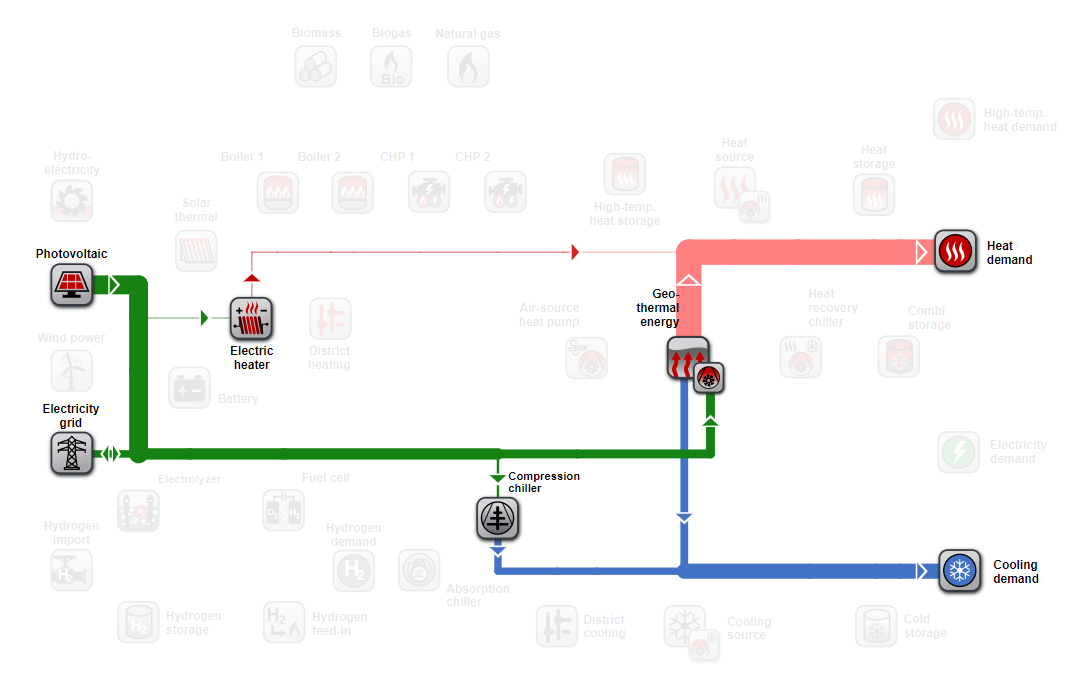Planning tool for buildings & districts
FAQ New

# Self-sufficiency (autarky) and self-consumption rate

For electricity-based energy systems, the degree of self-sufficiency (autarky) and the self-consumption rate are determined in the nPro tool. The definition of the two key figures can be found on this page.

## What is the definition of the self-sufficiency rate (autarky rate)?

The self-sufficiency rate indicates the proportion of electricity demand that is covered by self-generated electricity (e.g. from renewable energies or CHP units). The self-sufficiency rate $$S$$ in nPro refers to the generation and consumption of electrical energy and is defined as follows: $$S = 1-\frac{W_\text{import}}{W_\text{self-used}}$$ Here, $$W_\text{import}$$ describes the annual amount of electricity imported from the public grid and $$W_\text{self-used}$$ the amount of electricity consumed by the system itself, e.g. by heat pumps or to cover the electricity demand.Figure 1: System with high self-sufficiency rate and low self-consumption rate.

## What is the definition of the self-consumption rate?

The self-consumption rate indicates the proportion of self-generated electricity (e.g. from renewable energies or CHP units) used to cover the electricity demands. The self-consumption rate $$C$$ is therefore defined in the nPro tool as follows: $$C = 1-\frac{W_\text{import}-W_\text{generated}}{W_\text{generated}}$$ Here, $$W_\text{import}$$ describes the annual amount of electricity imported from the public grid and $$W_\text{generated}$$ the amount of electricity generated by technologies of the system. Technologies that can generate electricity are renewable energies (photovoltaics, wind power, hydroelectric power), combined heat and power (CHP) units and fuel cells.Figure 2: System with low self-sufficiency rate and high self-consumption rate.
In the nPro tool, the degree of self-sufficiency/autarky and the self-consumption rate are calculated for every system and can be used to evaluate the system performance.

## This might also interest you

### nPro Webtool

Plan your energy system with nPro!